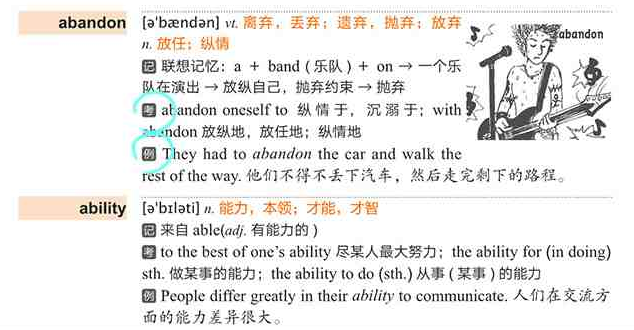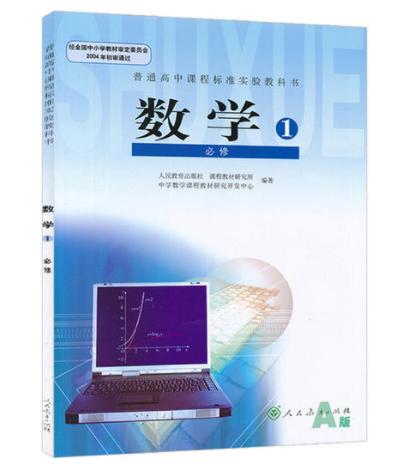# STL 库简介

## 标准模板库

C++ Standard Template Library，STL (STL 库)是泛型程序设计最成功应用的实例，STL 库最初由惠普实验室开发，于 1998 年被定为国际标准，正式成为 C++ 程序库的一部分。STL 库主要由 2 部分组成，其一是容器，包括了一些常用数据结构，第二部分是操作这些容器的泛型算法（如排序、查找）的模板的集合。
STL 库主要由 Alex Stepanov 主持开发，于 1998 年被加入 C++ 标准。STL 是由开发者精心设计的高质量的工具，不仅拥有更高的运行效率，也比一般的代码来得更安全。当开发者需要使用一些数据结构时可以直接用 STL 的容器，这样就可以跳过反复造轮子的过程，直接用上高效而安全的数据结构了。

# vector 容器

vector 是可变长的动态数组，可以存放任意数据类型和对象，是一种顺序容器。vector 容器经常用来构造顺序表，支持随机访问迭代器，且所有 STL 算法都能对 vector 进行操作。vector 容器使用连续的内存来存放元素，且和数组一样支持随机访问，如果在非头尾的位置插入或删除数据的效率较低。vector 容器的存储空间是自动维护的，当往 vector 填充数据元素时，vector 容器往往会分配比所需空间更大的存储空间，以便于开发者向容器中填充新的数据。当存储空间不足以存储数据时，vector 会自动以 2 倍的大小进行扩容，相比于限定存储大小且需要时刻提防越界的数组而言，vector 具有更强大的功能。

#include <vector>


## 定义 vector 容器

vector<数据类型名> 变量名


vector<int> ivec;


vector<int> ivec(50);    //容器大小为 50，int 默认值为 0


vector<int> ivec(50, -1);    //容器大小为 50，填充 -1


int iarray = {1, 2, 3, 4, 5};
vector<int> ivec(iarray, iarray + 5);


## vector 操作

vector 是可变长的动态数组，可以支持类似数组的用中括号进行随机访问的操作，也可以用这种方式进行数据修改。vector 容器常用的方法有：

void push_back(const T& x) 向量尾部增加一个元素 X
bool empty() const 判断向量是否为空，若为空，则向量中无元素
int size() const 返回容器中元素的个数
void clear() 清空容器中所有元素

#include <iostream>
#include <vector>
using namespace std;
int main()
{
vector<int> ivec;    //定义一个空的 vector<int> 容器

ivec.push_back(1);    //在容器末尾插入 3 个数字
ivec.push_back(2);
ivec.push_back(3);
cout << "ivec 中有"<< ivec.size() << "个元素，分别是：";
for(int i = 0; i < ivec.size(); i++)    //遍历容器
{
cout << ivec[i] << " ";
}
cout << endl;

ivec = 3;    //修改其中一个元素
cout << "ivec 的第一个元素是："<< ivec << endl;
ivec.clear();    //清空容器
cout << "ivec 中有"<< ivec.size() << "个元素" << endl;
if(ivec.empty() == true)
{
cout << "ivec 容器没有存储任何数据" << endl;
}
return 0;
}


ivec 中有3个元素，分别是：1 2 3
ivec 的第一个元素是：3
ivec 中有0个元素
ivec 容器没有存储任何数据


# 迭代器 Iterator

vector<数据类型名>::iterator 变量名;


vector 容器可以和数组一样用中括号加下标访问变量，为什么还需要有迭代器？这是因为迭代器不仅可以用来遍历，也可以结合函数支持插入数据、删除数据等操作，而且迭代器也可以用于遍历非顺序容器。vector 容器和通过迭代器进行工作的函数有：

iterator begin() 返回容器指向第一个元素的迭代器
iterator end() 返回指向最后一个元素的下一个位置的迭代器
iterator insert(iterator it,const T& x) 向量中迭代器指向元素前增加一个元素 x
iterator erase(iterator it) 删除向量中迭代器指向元素

vector 容器使用迭代器操作的简单样例如下：

#include <iostream>
#include <vector>
using namespace std;
int main()
{
vector<int> ivec;    //定义一个空的 vector<int> 容器
vector<int>::iterator it;    //定义一个 vector<int> 迭代器

ivec.push_back(1);    //在容器末尾插入 3 个数字
ivec.push_back(2);
ivec.push_back(3);
for(it = ivec.begin(); it < ivec.end(); it++)    //遍历容器
{
cout << *it << " ";
}
cout << endl;

it = ivec.begin();
ivec.insert(it, 3);    //在迭代器处插入数据 3
it = ivec.end() - 1;
ivec.erase(it);    //删除迭代器指向的数据
for(it = ivec.begin(); it < ivec.end(); it++)    //遍历容器
{
cout << *it << " ";
}
cout << endl;

return 0;
}


1 2 3
3 1 2


# list 容器

list 容器是双向链表容器，是以双向链表的形式实现的一种顺序型容器。由于 list 容器在底层是以链表的形式实现，因此容器中的元素并不存储于连续的内存空间中，对于已知的任何位置都能做到快速插入或删除元素。list 容器并不能像 vector 容器和数组那样支持随机访问，也不能用中括号访问数据，想要获取其中的某个数据就必须遍历。由于 list 容器实现的是双向链表，因此容器中任何一个元素访问其前驱和后继都很方便。若在实际情况下不需要对容器进行随机访问，且容器将会进行大量添加或删除元素的操作，建议使用 list 容器来存储。

#include <list>


## 定义 list 容器

list<数据类型名> 变量名


list<int> ilist;


list<int> ilist(50);    //容器大小为 50，int 默认值为 0


list<int> ilist(50, -1);    //容器大小为 50，填充 -1


list<int> ilist1(50, -1);
list<int> ilist2(ilist1);


## list 操作

list 容器并不是基于顺序表的实现，因此不支持类似数组的用中括号进行随机访问的操作。list 容器常用的方法有：

void push_back(const T& x) 容器尾部增加一个元素 X
void pop_back() 删除容器最后面的元素
void push_front(const T & x) 将 x 插入链表最前面
void pop_front() 删除容器最前面的元素
bool empty() const 判断向量是否为空，若为空，则向量中无元素
int size() const 返回容器中元素的个数
void clear() 清空容器中所有元素
void remove (const T & x) 删除和 x 相等的元素
void sort() 对容器进行快速排序
iterator begin() 返回容器指向第一个元素的迭代器
iterator end() 返回指向最后一个元素的下一个位置的迭代器
iterator insert(iterator it,const T& x) 向量中迭代器指向元素前增加一个元素 x
iterator erase(iterator it) 删除向量中迭代器指向元素

#include <iostream>
#include <list>
using namespace std;
int main()
{
list<int> ilist;    //定义一个空的 list<int> 容器
list<int>::iterator it;    //定义一个 vector<int> 迭代器

ilist.push_back(2);    //在容器末尾插入 3 个数字
ilist.push_back(3);
ilist.push_back(1);
ilist.sort();    //对容器中的元素排序

cout << "ilist 中有"<< ilist.size() << "个元素，分别是：";
for(it = ilist.begin(); it != ilist.end(); it++)    //遍历容器
{
cout << *it << " ";
}
cout << endl;

ilist.pop_front();    //删除容器第一个数据
ilist.push_front(5);    //在表头插入元素 5
ilist.pop_back();    //删除容器最后一个数据
ilist.remove(2);    //删除容器中的数据 2
ilist.insert(ilist.begin(), 4);    //在迭代器处插入数据 4
cout << "ilist 中有"<< ilist.size() << "个元素，分别是：";
for(it = ilist.begin(); it != ilist.end(); it++)    //遍历容器
{
cout << *it << " ";
}
cout << endl;

ilist.clear();    //清空容器
cout << "ilist 中有"<< ilist.size() << "个元素" << endl;
if(ilist.empty() == true)
{
cout << "ilist 容器没有存储任何数据" << endl;
}
return 0;
}


ilist 中有3个元素，分别是：1 2 3
ilist 中有2个元素，分别是：4 5
ilist 中有0个元素
ilist 容器没有存储任何数据


# stack 和 queue

## stack 容器

stack 是栈容器，从数据结构的角度上说栈是一种操作受限的线性表，访问、添加和删除元素都只能对栈顶进行操作。栈内的元素是不允许访问的，要访问栈内的元素就只能将其上方的元素出栈，使之变成新的栈顶。

#include <stack>


stack<数据类型名> 变量名


void pop() 栈顶元素出栈
T & top() 返回栈顶元素的引用
void push (const T & x) 元素 x 入栈
bool empty() const 判断容器是否为空
int size() const 返回容器中元素的个数

#include <iostream>
#include <stack>
using namespace std;
int main()
{
stack<int> ist;    //定义一个空的 stack<int> 容器

ist.push(1);    //3 个数据入栈
ist.push(2);
ist.push(3);
cout << "ist 中有"<< ist.size() << "个元素，分别是：";
while(ist.empty() == false)    //循环直到栈空为止
{
cout << ist.top() << " ";    //输出栈顶元素
ist.pop();    //栈顶元素出栈
}
cout << endl;

return 0;
}


ist 中有3个元素，分别是：3 2 1


## queue 容器

queue 是队列容器，从数据结构的角度上说队列也是一种操作受限的线性表，只能访问队列头和队列尾的元素，添加和删除元素分别只能对队列尾和队列头进行操作。队列内的元素是不允许访问的，要访问队列内的元素就只能将队列头的元素出队列，使之变成新的队列头。

#include <queue>


queue<数据类型名> 变量名


void pop() 队列头元素出栈
T & front() 返回队列头元素的引用
T & back()() 返回队列尾元素的引用
void push (const T & x) 元素 x 入队列
bool empty() const 判断容器是否为空
int size() const 返回容器中元素的个数

#include <iostream>
#include <queue>
using namespace std;
int main()
{
queue<int> ique;    //定义一个空的 queue<int> 容器

ique.push(1);    //3 个数据入队列
ique.push(2);
ique.push(3);
cout << "ique 队列尾元素是：" << ique.back() << endl;
cout << "ique 中有"<< ique.size() << "个元素，分别是：";
while(ique.empty() == false)
{
cout << ique.front() << " ";    //输出队列头元素
ique.pop();    //队列头出队列
}
cout << endl;

return 0;
}


# map 容器

map 是映射容器，顾名思义是一种将某个数据元素映射到另一个数据元素的容器，是一种关联式容器。map 容器存储的都是 pair 对象，每组映射都由“键 (key)”和“值 (value)”组成的一组键值对，各个键值对的键和值可以是任意数据类型，包括 C++ 基本数据类型、使用结构体或类。选用 string 字符串作为键的类型作为索引，是 map 容器的一种常见的用法。使用 map 容器存储的各个键值对中，键的值既不能重复也不能被修改。

#include <map>


## 理解 map 容器## 定义 map 容器

map<数据类型名1, 数据类型名2> 变量名


map<string, int> words;
words["C++"] = 1;


map<string, int> words;
string tword;
while(cin >> tword)
{
words[tword]++;
}


## map 容器操作

map 容器可以支持类似数组的用中括号进行随机访问的操作，只不过中括号内提供的是 key 而非下标，也可以用这种方式进行数据修改。map 容器常用的方法有：

bool empty() const 判断向量是否为空，若为空，则向量中无元素
int size() const 返回容器中元素的个数
void clear() 清空容器中所有元素
int count(key) 在容器中，查找键为 key 的键值对的个数
iterator find(key) 在容器中查找键为 key 的键值对，找到返回指向该键值对的迭代器，反之返回和 end()
iterator begin() 返回容器指向第一个元素的迭代器
iterator end() 返回指向最后一个元素的下一个位置的迭代器
iterator erase(iterator it) 删除向量中迭代器指向元素

#include <iostream>
#include <map>
#include <string>
using namespace std;
int main()
{
map<string, int> words;
map<string, int>::iterator it;

words["Python"] = 3;    //构造 3 组映射
words["Java"] = 2;
words["C++"]++;
cout << "map 容器中有" << words.size() << "对映射，分别是：" << endl;
for(it = words.begin(); it != words.end(); it++)
{
cout << it->first << " -> " << it->second << endl;
}

it = words.find("C++");    //查找 key C++
if(it != words.end())
{
cout << "key：C++ 在容器中" << endl;
}
if(words.count("C") == 0)    //利用 count 判断 key C 是否存在
{
cout << "key：C 不在容器中" << endl;
}

words.clear();    //清空容器
cout << "words 中有"<< words.size() << "个元素" << endl;
if(words.empty() == true)
{
cout << "words 容器没有存储任何数据" << endl;
}

return 0;
}


map 容器中有3对映射，分别是：
C++ -> 1
Java -> 2
Python -> 3
key：C++ 在容器中
key：C 不在容器中
words 中有0个元素
words 容器没有存储任何数据


# set 容器

set 集合容器也是一种关联式容器，容器中仅存储一组 key。集合容器符合数学对集合的定义，这里复习一下数学必修一的知识，即集合容器具有确定性、互异性和无序性。简而言之，集合容器是一种不重复元素的序列结构。如果你不需要对一组数据进行统计，而是仅需要记录出现过哪些数据，可以使用 set 容器进行存储。#include <set>


set 容器常用于判断数据的存在性，一些常用的方法如下：

bool empty() const 判断向量是否为空，若为空，则向量中无元素
int size() const 返回容器中元素的个数
void clear() 清空容器中所有元素
int count(key) 在容器中，查找键为 key 的键值对的个数
iterator find(key) 在容器中查找键为 key 的键值对，找到返回指向该键值对的迭代器，反之返回和 end()
iterator begin() 返回容器指向第一个元素的迭代器
iterator end() 返回指向最后一个元素的下一个位置的迭代器
iterator insert(const T& x) 容器中增加一个元素 x
iterator erase(iterator it) 删除容器中迭代器指向元素

#include <iostream>
#include <set>
using namespace std;
int main()
{
set<int> iset;
set<int>::iterator it;

iset.insert(1);    //向集合中放入 3 个 key
iset.insert(2);
iset.insert(3);
iset.insert(4);
iset.erase(4);    //删除元素 4
cout << "iset 中有"<< iset.size() << "个元素，分别是：";
for(it = iset.begin(); it != iset.end(); it++)    //遍历容器
{
cout << *it << " ";
}
cout << endl;

it = iset.find(1);    //查找数据 1
if(it != iset.end())
{
cout << "数据 1 在容器中" << endl;
}
if(iset.count(4) == 0)    //利用 count 判断 4 是否存在
{
cout << "数据 4 不在容器中" << endl;
}

iset.clear();    //清空容器
cout << "iset 中有"<< iset.size() << "个元素" << endl;
if(iset.empty() == true)
{
cout << "iset 容器没有存储任何数据" << endl;
}

return 0;
}


iset 中有3个元素，分别是：1 2 3

iset 中有0个元素
iset 容器没有存储任何数据


# String 类

#include <string>


## String 初始化

String 类有很多的构造函数，常用的构造函数有：

string(const char *str) 返回 String 对象，用字符串 str 初始化
string(int n,char ch) 返回 String 对象，用 n 个字符 ch 初始化

#include <iostream>
#include <string>
using namespace std;
int main()
{
string str1 = "Hello,";    //直接赋值
string str2("world");    //构造函数，用常量初始化
string str3(3,'!');    //构造函数，3个连续的字符'!'

cout << str1 << str2 << str3 << endl;
//输出“Hello,world!!!”

return 0;
}


## String 操作

string &assign(const string &str) 把 str 赋值给 String 对象
string &append(const string &str) 把 str 连接到当前 String 对象结尾
int compare(const string &str) const 比较当前 String 对象和 str 的大小
void swap(string &str) 交换当前 String 对象和 str 的值
int size()const 返回当前 String 对象的大小
int length()const 返回当前 String 对象的长度
bool empty()const 判断当前 String 对象是否为空

#include <iostream>
#include <string>
using namespace std;
int main()
{
string str1;
string str2("world");    //构造函数，用常量初始化
string str3(3,'!');    //构造函数，3个连续的字符'!'
string str4;
string str5 = "PHP is the best language ever, ever.";

if(str1.empty() == true)
{
cout << "此时 str1 为空，请输入：";
}
cin >> str1;    //输入“Hello,”
str4.assign(str1);    //将 str1 赋值给 str4
str4.append(str2);    // 将 str2 接到 str4 末尾
str4 += str3;    // 将 str3 接到 str4 末尾
cout << "str4 有 " << str4.size() << "个字符，它的内容是：" + str4 << endl;
str5.swap(str4);    //交换 str4 和 str5
cout << "str4 有 " << str4.length() << "个字符，它的内容是：" + str4 << endl;
if(str4.compare(str5) == 1)
{
cout << "str4 大于 str5" << endl;
}

return 0;
}


# 参考资料

posted @ 2021-02-28 22:06  乌漆WhiteMoon  阅读(1225)  评论(0编辑  收藏  举报